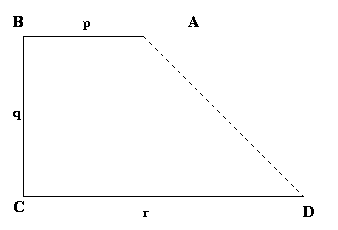#### You may also like### Two Cubes

Two cubes, each with integral side lengths, have a combined volume equal to the total of the lengths of their edges. How big are the cubes? [If you find a result by 'trial and error' you'll need to prove you have found all possible solutions.]### Rationals Between...

What fractions can you find between the square roots of 65 and 67?### Square Mean

Is the mean of the squares of two numbers greater than, or less than, the square of their means?

# Biggest Enclosure

##### Age 14 to 16Challenge Level

Three fences of lengths $p$, $q$ and $r$ with $p< q< r$ are arranged to form three sides $AB$, $BC$ and $CD$ of a field $ABCD$ with right angles at $B$ and $C$.The diagram shows one possibility but the fences can be exchanged to make different enclosures.

The enclosure is completed by joining $A$ and $D$.

How should the rods be arranged to make the area of the enclosure as big as possible?